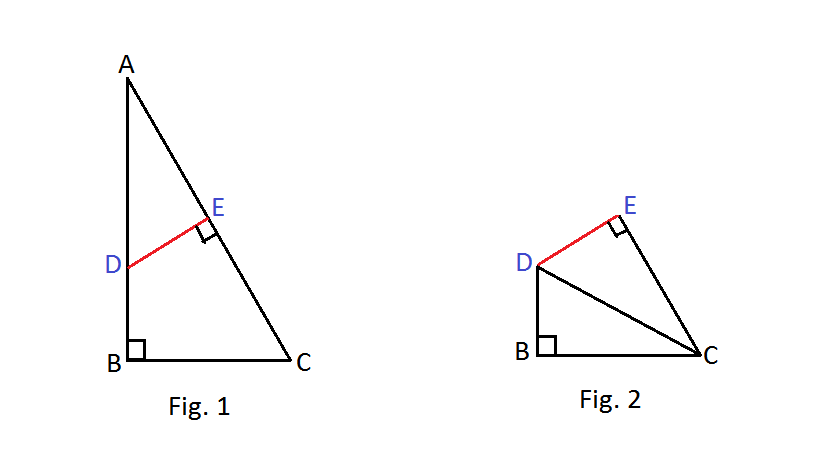# Simple as ABC!

Geometry Level 3A right angled triangle $ABC$ (non-isosceles) was cut from a piece of paper as shown in Fig.1.

A point $E$ was taken at the mid-point of hypotenuse and the figure was folded along $DE$ such that $A$ coincides with $C$. As a result, two separate right triangles $CBD$ and $CED$ were formed. (Fig. 2)

Take $AC = h$, $AB = p$, $BC = b$.

Which of these answer choices is equal to the ratio $\dfrac{ AC}{CD}$?

×